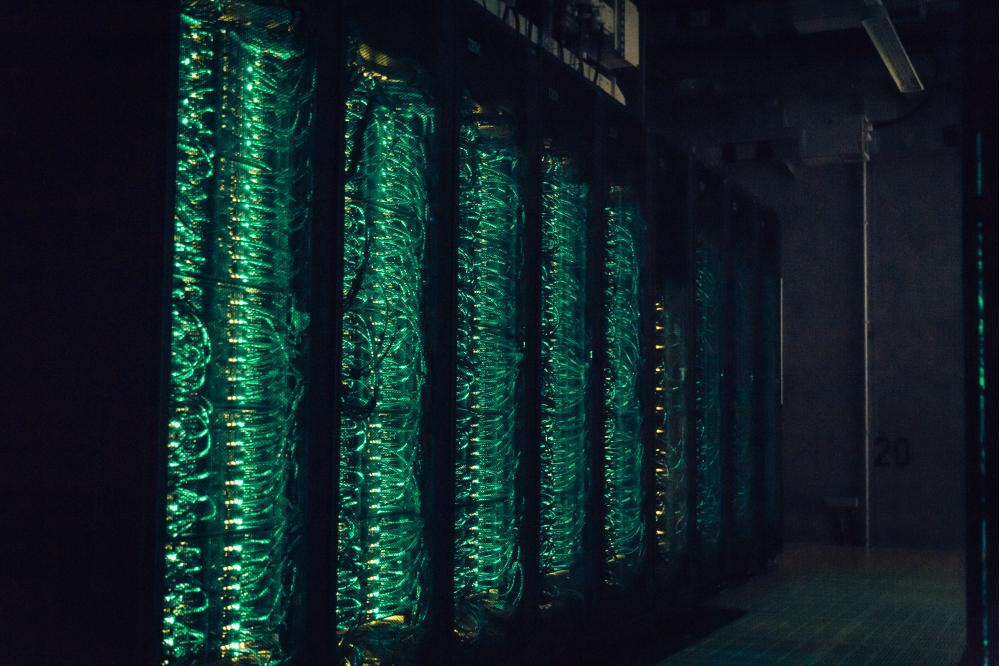# Gluonic Green‘s Functions of Quantum ChromodynamicsDefault Image - ProjectHessen Agentur/Jürgen Kneifel

## Introduction

According to modern physics, nature can be explained by four fundamental forces: the gravitational, the electromagnetic, the weak and the strong force. Quantum chromodynamics (QCD) is the contemporary theory of the strong interaction and describes the interaction of quarks and gluons. Although QCD was formulated in the 1970s, many aspects still have to be explored.

## Methods

While so-called perturbation theory provides a very good description at high energies, it cannot be applied at low energies. To access the low energy regime of this quantum field theory, non-perturbative methods have been developed. Some of these methods demand a very large amount of computing power, and high performance computing allows performing numerical calculations that were not feasible until recently.

Dyson-Schwinger equations (DSEs) are a practical tool for non-perturbative calculations. One possible application is the calculation of the elementary Green's functions of the strong force which describes the interaction of quarks. Green's functions can be considered the elementary building blocks of a quantum field theory and the Dyson-Schwinger equations are their equations of motion. An aspect is that for the strong force Green's functions are not physical objects themselves in the sense that they cannot be measured directly. However, they are the input needed to calculate physical quantities like the mass and form factors of the proton.

## Results

In this project we have overcome technical limitations in the solution of the DSEs for the gluonic two-point functions in Landau-gauge QCD, i.e. the gluon and ghost propagators. We have for the first time included dynamical 3-point vertexfunctions selfconsistently in the solutions of the coupled non-linear integral equations that are obtained from truncating the infinite tower of DSEs in the spirit vertex expansions. [2,3,4,5] Our fully iterated solution to a system of DSEs that is complete on the level of 3-point functions, in comparison to lattice data and previous results for the propagators, for the first time demonstrated that these kinds of vertex expansions are feasible and it provided encouraging evidence for their convergence.

## Discussion

Vertex functions also are needed for a wide range of phenomenological studies in hadron physics. Thus, to calculate how these vertices behave at low energies enables more precise calculations of hadronic observables. In (Cyrol et al.) we have calculated this four-gluon vertex from its DSE. Our results are: First, a self-consistent solution of four-gluon vertex Dyson-Schwinger equation did not exist before and it was not clear if a solution in the suggested truncation scheme was possible at all. Secondly, we have calculated the full momentum dependence. This allows employing the obtained results to study the influence of the fourgluon vertex on other scattering processes. Furthermore, we have gathered promising evidence that the full four-gluon vertex can be approximated rather well by a single quantity. This will alleviate future studies significantly. To calculate the four-gluon vertex, it is necessary to know the non-perturbative behavior of the propagators and the three-gluon vertex. We used results from previous Dyson-Schwinger studies.[2,3,4] However, these results depend on the four-gluon vertex.

## Outlook

Now we want to combine all these separate studies to obtain a self-contained and self-consistent description of the propagators and the vertices. The first important step to achieve that is documented in a master thesis.

## Last Update

• Last Update: 2020-09-28 18:30